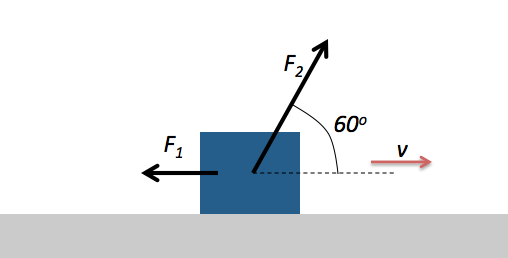Classical Mechanics

# Power input to a systemThe figure above shows constant forces $$\vec{F_1}$$ and $$\vec{F_2}$$ acting on a box as the box slides rightward across a frictionless horizontal floor. Force $$\vec{F_1}$$ acts horizontally leftward with a magnitude of $$4 \text{ N} .$$ Force $$\vec{F_2}$$ is applied in a direction that makes a $$60^\circ$$ angle with the floor with a magnitude of $$8 \text{ N}.$$ The speed $$v$$ of the box at a certain instant is $$6 \text{ m/s}.$$ What is the net power acting on the box?

A skier is pulled by a towrope up a frictionless ski slope that makes a $$30^ \circ$$ angle with the horizon. The rope moves parallel to the slope at a constant speed of $$1 \text{ m/s}.$$ The rope does $$800 \text{ J}$$ of work to the skier as the skier moves a distance of $$8 \text{ m}$$ up the incline. If instead the rope moved at a constant speed of $$2 \text{ m/s}$$, while the skier moves the same distance, with how much power would the rope do work to the skier?

A $$4 \text{ kg}$$ cactus initially at rest accelerates uniformly for $$16$$ seconds. If the cactus' speed after the acceleration is $$16 \text{ m/s} ,$$ what is the instantaneous power with which work is done to the cactus, just before the end of its acceleration?

A freight elevator is loaded with a cab with a total mass of $$1200 \text{ kg},$$ which is required to travel upward $$18 \text{ m}$$ in $$100$$ seconds, starting and ending at rest. The elevator's counterweight has a mass of only $$1000 \text{ kg},$$ so the elevator motor must help. With how much power must the motor do work to the cab?

The gravitational acceleration is $$g= 10 \text{ m/s}^2.$$

A $$80 \text{ kg}$$ block is pulled at constant speed of $$7.0 \text{ m/s}$$ across a horizontal floor by an applied force of magnitude $$4 \text{ N}$$ in a direction that makes a $$60 ^\circ$$ angle with the floor. With how much power does the force do work on the block?

×